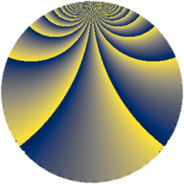# Properties

 Label 1110.2.nLevel $1110$ Weight $2$ Character orbit 1110.n Rep. character $\chi_{1110}(443,\cdot)$ Character field $\Q(\zeta_{4})$ Dimension $152$ Sturm bound $456$

# Related objects

## Defining parameters

 Level: $$N$$ $$=$$ $$1110 = 2 \cdot 3 \cdot 5 \cdot 37$$ Weight: $$k$$ $$=$$ $$2$$ Character orbit: $$[\chi]$$ $$=$$ 1110.n (of order $$4$$ and degree $$2$$) Character conductor: $$\operatorname{cond}(\chi)$$ $$=$$ $$555$$ Character field: $$\Q(i)$$ Sturm bound: $$456$$

## Dimensions

The following table gives the dimensions of various subspaces of $$M_{2}(1110, [\chi])$$.

Total New Old
Modular forms 472 152 320
Cusp forms 440 152 288
Eisenstein series 32 0 32

## Trace form

 $$152q + 8q^{3} + O(q^{10})$$ $$152q + 8q^{3} + 8q^{12} - 152q^{16} - 64q^{25} - 16q^{27} - 24q^{30} - 8q^{33} + 24q^{37} + 16q^{40} + 16q^{46} - 8q^{48} + 16q^{58} - 16q^{67} - 16q^{70} + 16q^{73} + 80q^{75} - 20q^{78} + 144q^{81} - 96q^{85} - 96q^{90} + O(q^{100})$$

## Decomposition of $$S_{2}^{\mathrm{new}}(1110, [\chi])$$ into newform subspaces

The newforms in this space have not yet been added to the LMFDB.

## Decomposition of $$S_{2}^{\mathrm{old}}(1110, [\chi])$$ into lower level spaces

$$S_{2}^{\mathrm{old}}(1110, [\chi]) \cong$$ $$S_{2}^{\mathrm{new}}(555, [\chi])$$$$^{\oplus 2}$$